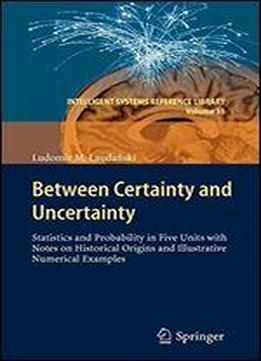# Between Certainty And Uncertainty: Statistics And Probability In Five Units With Notes On Historical Origins And Illustrative Numerical Examples by Ludomir M. LaudaЕ„ski / 2012 / English / PDF

Between Certainty & Uncertainty is a one-ofa-kind short course on statistics for students, engineers and researchers. It is a fascinating introduction to statistics and probability with notes on historical origins and 80 illustrative numerical examples organized in the five units: Chapter 1 Descriptive Statistics: Compressing small samples, basic averages - mean and variance, their main properties including Gods proof linear transformations and z-scored statistics . Chapter 2 Grouped data: Udny Yules concept of qualitative and quantitative variables. Grouping these two kinds of data. Graphical tools. Combinatorial rules and qualitative variables. Designing frequency histogram. Direct and coded evaluation of quantitative data. Significance of percentiles. Chapter 3 Regression and correlation: Geometrical distance and equivalent distances in two orthogonal directions as a prerequisite to the concept of two regression lines. Misleading in interpreting two regression lines. Derivation of the two regression lines. Was Hubble right? Houbolts cloud. What in fact measures the correlation coefficient? Chapter 4 Binomial distribution: Middle ages origins of the binomials figurate numbers and combinatorial rules. Pascals Arithmetical Triangle. Bernoullis or Poisson Trials? John Arbuthnot curing binomials. How Newton taught S. Pepys probability. Jacob Bernoullis Weak Law of Large Numbers and others. Chapter 5 Normal distribution and binomial heritage Tables of the normal distribution. Abraham de Moivre and the second theorem of de Moivre-Laplace. Chapter 1 Descriptive Statistics: Compressing small samples, basic averages - mean and variance, their main properties including Gods proof linear transformations and z-scored statistics . Chapter 2 Grouped data: Udny Yules concept of qualitative and quantitative variables. Grouping these two kinds of data. Graphical tools. Combinatorial rules and qualitative variables. Designing frequency histogram. Direct and coded evaluation of quantitative data. Significance of percentiles. Chapter 3 Regression and correlation: Geometrical distance and equivalent distances in two orthogonal directions as a prerequisite to the concept of two regression lines. Misleading in interpreting two regression lines. Derivation of the two regression lines. Was Hubble right? Houbolts cloud. What in fact measures the correlation coefficient? Chapter 4 Binomial distribution: Middle ages origins of the binomials figurate numbers and combinatorial rules. Pascals Arithmetical Triangle. Bernoullis or Poisson Trials? John Arbuthnot curing binomials. How Newton taught S. Pepys probability. Jacob Bernoullis Weak Law of Large Numbers and others. Chapter 5 Normal distribution and binomial heritage Tables of the normal distribution. Abraham de Moivre and the second theorem of de Moivre-Laplace. Chapter 5 Normal distribution and binomial heritage Tables of the normal distribution. Abraham de Moivre and the second theorem of de Moivre-Laplace.

views: 207